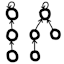#Disjoint-set data structure

## Introduction

The disjoint-set data structure, also known as union-find, is useful for quickly testing whether nodes in a graph are connected or not. The code provided here implements union-by-size, path compression, and size queries. My high-quality implementations are open-source and are available in a number of programming languages.

## Documentation

Here is the API documentation of the Java version of the library: (other languages are largely similar)

`class DisjointSet`

Represents a set of disjoint sets. Also known as the union-find data structure. Main operations are querying if two elements are in the same set, and merging two sets together. Useful for testing graph connectivity, and is used in Kruskal’s algorithm.

`DisjointSet(int numElems)`

Constructs a new set containing the given number of singleton sets. For example, new DisjointSet(3) → {{0}, {1}, {2}}.

`boolean areInSameSet(int elemIndex0, int elemIndex1)`

Tests whether the given two elements are members of the same set. Note that the arguments are orderless.

`boolean mergeSets(int elemIndex0, int elemIndex1)`

Merges together the sets that the given two elements belong to. This method is also known as “union” in the literature. If the two elements belong to different sets, then the two sets are merged and the method returns `true`. Otherwise they belong in the same set, nothing is changed and the method returns `false`. Note that the arguments are orderless.

`int getNumElements()`

Returns the number of elements among the set of disjoint sets; this was the number passed into the constructor and is constant for the lifetime of the object. All the other methods require the argument `elemIndex` to satisfy 0 ≤ `elemIndex` < `getNumElements()`.

`int getNumSets()`

Returns the number of disjoint sets overall. This number decreases monotonically as time progresses; each call to `mergeSets()` either decrements the number by one or leaves it unchanged. 0 ≤ result ≤ `getNumElements()`.

`int getSizeOfSet(int elemIndex)`

Returns the size of the set that the given element is a member of. 1 ≤ result ≤ `getNumElements()`.

## Source codeJava (SE 5+)
• DisjointSet.java: Data structure library
• DisjointSetTest.java: JUnit test suite
• SimpleDisjointSet.java: An easier-to-understand version of DisjointSet for educational purposes. Less efficient because it creates a node object per element, instead of using flat arrays of integers. But it has the same asymptotic time complexity as the streamlined version. This is not the same as the NaiveDisjointSet within DisjointSetTest, which has much worse asymptotic time complexity (but is easy to verify for correctness).
TypeScript / JavaScript
Python
C++ (C++11 and above)
C (C99 and above)
C#
Rust